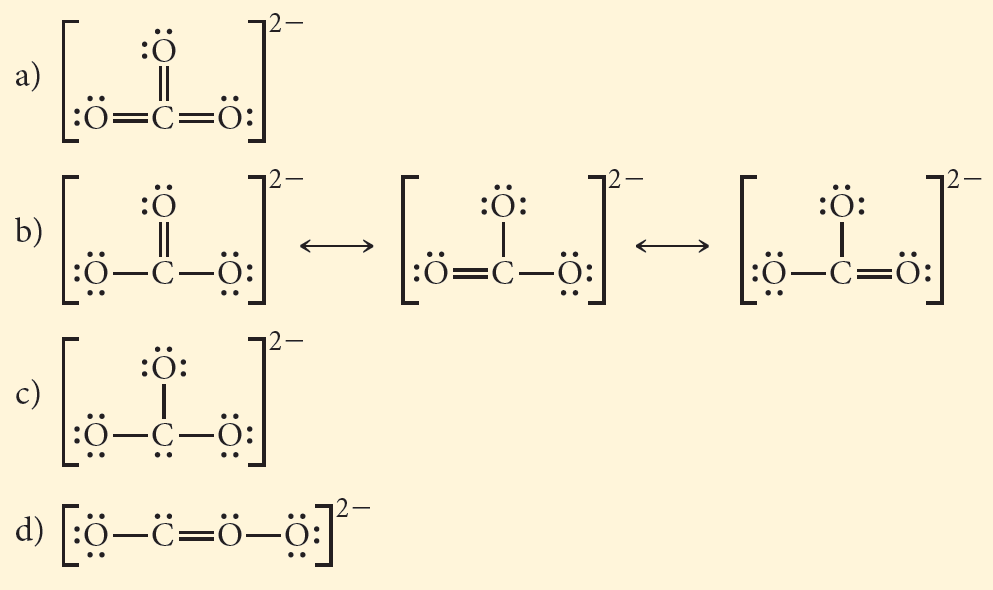# Problem: Which is the correct Lewis structure for CO32–?

🤓 Based on our data, we think this question is relevant for Professor Bridson's class at MEMPHIS.

###### FREE Expert Solution

(1) C → less electronegative than O → C is central atom

(2) Inspecting the formal charges in each bond possible

non-bonding electron = lone pair e-

Cl

▪ Formal Charge:

F. C. = 7 – (5 + 2)
F. C. = 7 – 7
F. C. = 0

O (single bond)

▪ Formal Charge:

F. C. = 6 – (1 + 6)
F. C. = 6 – 7
F. C. = -1

O (double bond)

▪ Formal Charge:

F. C. = 6 – (2 + 4)
F. C. = 6 – 6
F. C. = 0###### Problem Details

Which is the correct Lewis structure for CO32–?